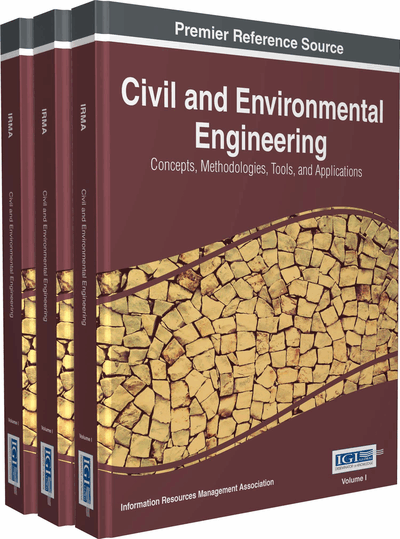# Application of Artificial Neural Network and Genetic Programming in Civil Engineering

Pijush Samui, Dhruvan Choubisa, Akash Sharda
DOI: 10.4018/978-1-4666-9619-8.ch044
OnDemand:
(Individual Chapters)
Available
\$37.50
No Current Special Offers

## Abstract

This chapter examines the capability of Genetic Programming (GP) and different Artificial Neural Network (ANN) (Backpropagation [BP] and Generalized Regression Neural Network [GRNN]) models for prediction of air entrainment rate (QA) of triangular sharp-crested weir. The basic principal of GP has been taken from the concept of Genetic Algorithm (GA). Discharge (Q), drop height (h), and angle in triangular sharp-crested weir (?) are considered as inputs of BP, GRNN, and GP. Coefficient of Correlation (R) has been used to assess the performance of developed GP, BP, and GRNN models. For a perfect model, the value of R should be close to one. A sensitivity analysis has been carried out to determine the effect of each input parameter. This chapter presents a comparative study between the developed BP, GRNN, and GP models.
Chapter Preview
Top

## Introduction

A weir is designed to change flow characteristics of river. Different shapes of weir are used such as rectangular weir, triangular or v-notch weir, the broad-crested weir, etc. The amount of dissolved oxygen in a river system is increased by weir. The change in oxygen concentration due to weir is given by the following equation

(1) where dm/dt is mass transfer rate of gas molecules across an interface, Cs and C are the saturation concentration of oxygen in water at prevailing ambient conditions and the actual concentration of oxygen in the water at time t-difference being proportional to the concentration gradient, kL is bulk liquid film coefficient, A is the air–water contact area and V is the volume of water associated with this. Ervine, et al. (1980) explained air entrainment mechanisms by weirs. They gave four mechanism of air entrainment.

So, the determination of air entrainment rate (QA) of triangular sharp-crested weir is an imperative task in civil engineering. Researchers determined air entrainment rate and aeration efficiency of different shapes weirs (Baylar & Bagatur, 2000; 2001a; 2001b; 2006; Baylar, et al., 2001a; 2001b; Baylar, 2002, 2003; Baylar & Emiroglu, 2007; Emiroglu & Baylar, 2003a; 2003b; 2005). Baylar, et al. (2008) successfully adopted Adaptive Neuro Fuzzy Inference System(ANFIS) for prediction of QA of triangular sharp-crested weir. ANFIS has been successfully used to model different problems in water (ASCE, 2000; Kisi, 2004a; 2004b; Hanbay, et al., 2006a; 2006b; 2007; Abolpour, et al., 2007; Baylar, et al., 2007). ANFIS can be trained to provide input/output data mappings and one can get the relationship between model inputs and corresponding outputs. It enables the knowledge that has been learnt in the network training to be translated into a set of fuzzy rules that describe the model input/output relationship in a more transparent fashion. ANFIS makes inference by fuzzy logic and shapes fuzzy membership function using neural network (Altrock, 1995; Brown & Harris, 1995).

However, the developed ANFIS did not give any equation for prediction of air entrainment rate and aeration efficiency of triangular sharp-crested weir.

## Complete Chapter List

Search this Book:
Reset